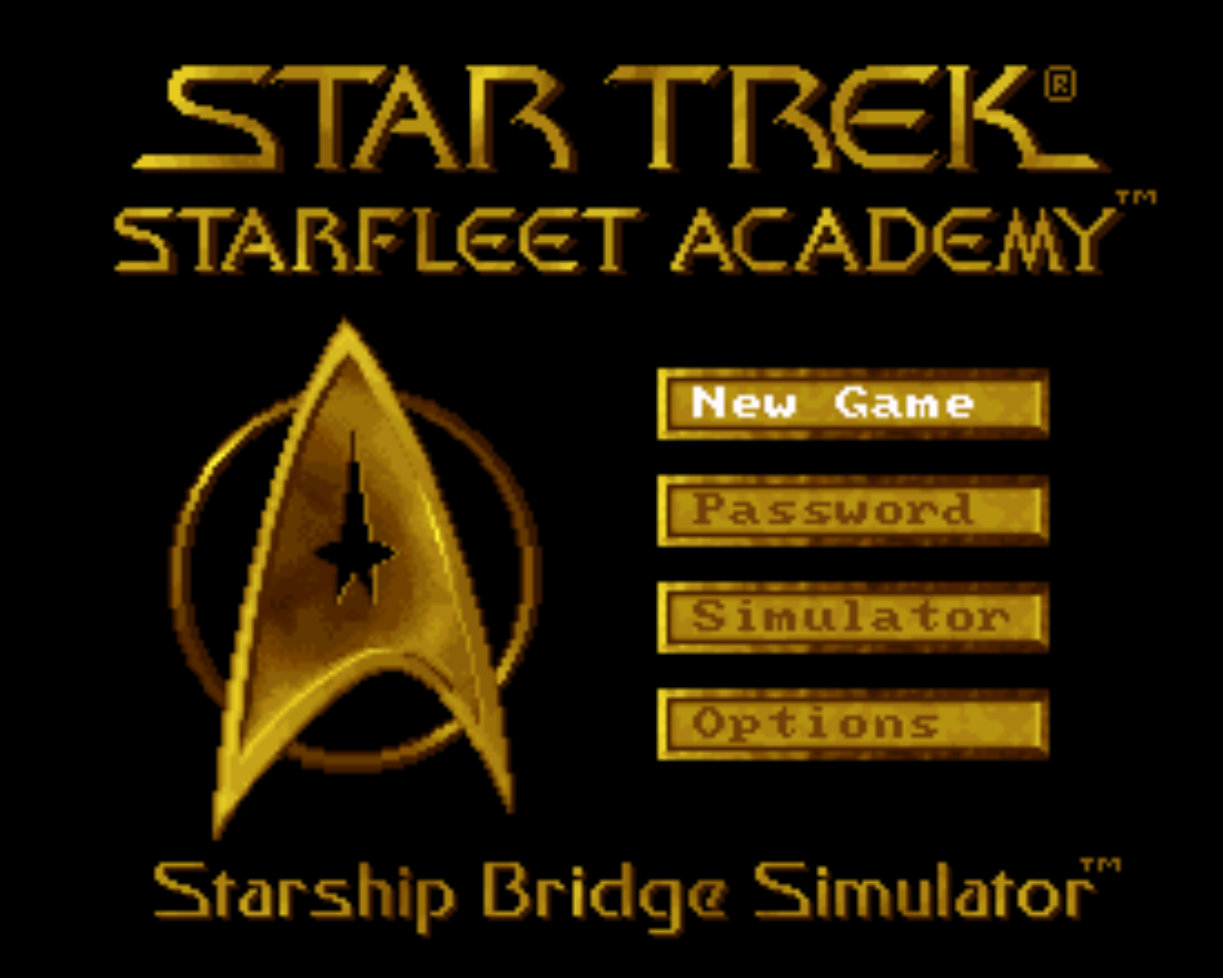Star Trek Academy: Starship Bridge Simulator Guides and Walkthroughs

This page here will share minor tidbits, tricks, cheats and hints about Star Trek Academy: Starship Bridge Simulator. As with many of the other guides on my website these are designed to be very google friendly. If you're stuck, and googling to get done a certain part of the game, that's hopefully how you found this guide!

Star Trek Academy: Starship Bridge Simulator Game Genie Codes (USA)

Star Trek Academy: Starship Bridge Simulator Pro Action Replay Codes (USA)Mission 101: X X X R X X Y R X R Y L

Mission 102: X X X R A X A L X R Y Y

Mission 103: X X X R L Y Y A X R Y X

Mission 104: X X X R Y Y A X X R Y L

Mission 105: X X X R B A X L X R Y A

Mission 201: X X X R R X Y R X Y Y B

Mission 202: X X X L X X A B X Y Y A

Mission 203: X X X L A Y Y A X Y Y A

Mission 204: X X X L L Y A X X Y Y X

Mission 205: X X X L Y A X L X Y Y A

Mission 301: X X X L B X Y R Y L X X

Mission 302: X X X L R X Y R Y L X R

Mission 303: X X X B X X A L Y L X B

Mission 304: X X X B A Y Y A Y L X A

Mission 305: X X X B L Y A X Y L X X

Mission 401: X X X B Y X Y R Y Y B L

Mission 402: X X X B B X A B Y Y B A

Mission 403: X X X B R Y Y A Y Y B X

Mission 404: X X X A X Y Y A Y Y B A

Mission 405: X X X A A Y A Y Y Y B B

Final Exam: X X X A L A X R Y Y B Y

Various Codes

Choose Star Trek Series Names: Press X, Y, X, Y at the New Cadet Registratio screen, hold down L, R, Select and press A, B, A, B.

New ships in Training Simulator: Hold down L, R, Select, and press A, Y, B, Y at any of the Ship Selection Menu.